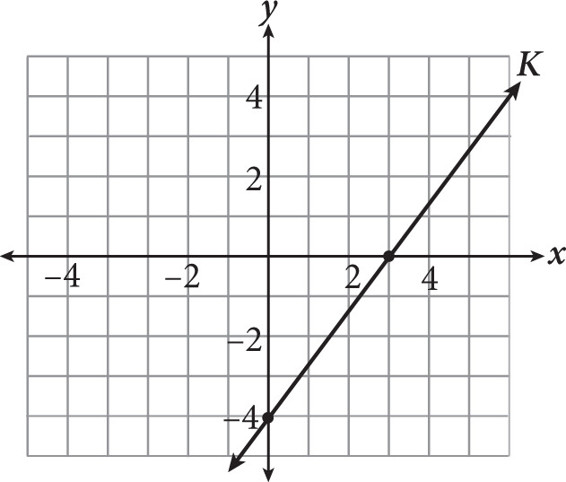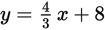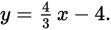# SAT Math Multiple Choice Question 295: Answer and Explanation

### Test Information

Question: 295

10.Where will the graph ofintersect line K shown above?

• A. (–3, 4)
• B. (9, 20)
• C. The graphs will never intersect.
• D. It is not possible to determine where the graphs will intersect because the y-intercept of the given line does not fit on the coordinate plane.

Getting to the Answer: Line K intersects the y-axis at -4. From there, it rises 4 units and runs 3 units to the next point, making its equationNow, you could set this equation equal to the one in the question and solve for x, or you could recognize that the two lines have the same slope but different y-intercepts, which means they are parallel lines and will never intersect.